### Home > CCA > Chapter Ch3 > Lesson 3.2.1 > Problem3-39

3-39.

For each of the shapes formed by algebra tiles below: Homework Help ✎

• Sketch and label the shape on your paper and write an expression that represents the perimeter.

• Simplify your perimeter expression as much as possible.

1.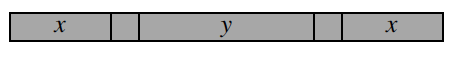• Start by labeling the sides.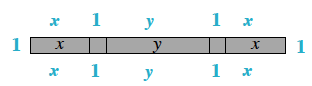$x+1+y+1+x+1+x+1+y+1+x+1$
Combine like terms.

$4x+2y+6$

1.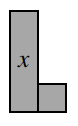• See help for part (a).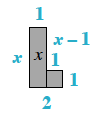$2x+4$

1.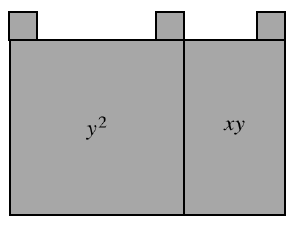• See help for part (a).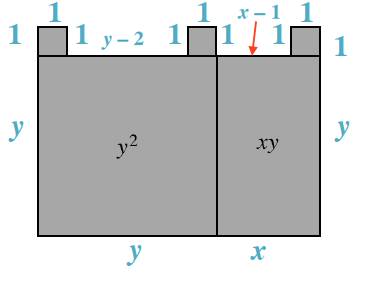$4y+2x+6$

1.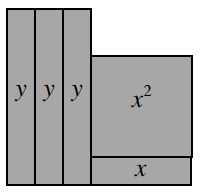• See help for part (a).

$2y+2x+6$